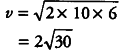# Shyam drops a ball of 100 g from a building of height 10 m

Shyam drops a ball of 100 g from a building of height 10 m. What will be its kinetic energy at the height of 4 m? What will happen to its total mechanical energy? Give reasons to justify your answer.

Given, mass, m = 100 g= 0.1 kg, height, h = 10 m
Potential energy, PE = mgh = 0.1 X10 X10 = 10J
When body reaches at height 6 m , in velocity is given by
{{v}^{2}} = {{u}^{2}} + 2gh
{{v}^{2}} = 0 + 2 x 10 x 6Now, its kinetic energy at this point is given by,
= 1/2 m\${{v}^{2}}\$ = 1/2 x 0.1 x 4 x 30 = 6 J
As, mechanical energy = KE + PE [at highest point]
Mechanical energy = 0+10 J = 10J
Mechanical energy at 4 m height
= KE+ PE= 6 + 0.l x 10x 4= 10J
Hence, it is seen that total mechanical energy always remains constant during the motion.